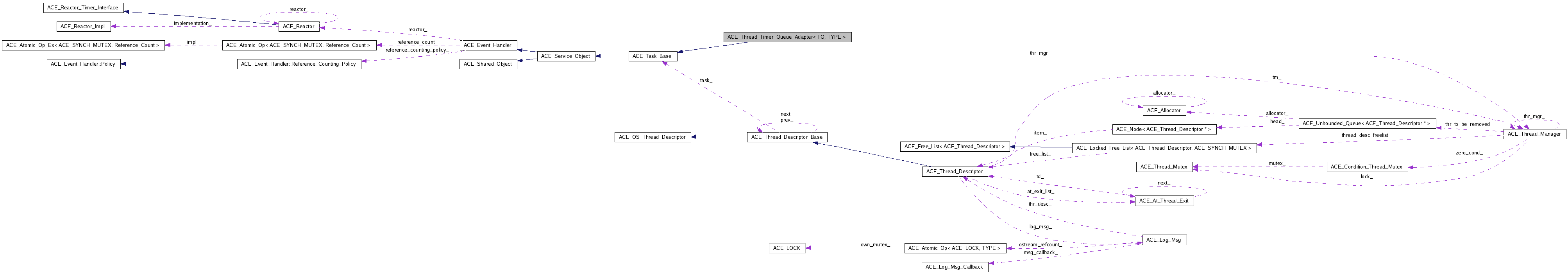Adapts an ACE timer queue using a separate thread for dispatching. More...

`#include <Timer_Queue_Adapters.h>`[legend][legend]

List of all members.

## Public Types

typedef TQ TIMER_QUEUE
Trait for the underlying queue type.

## Public Member Functions

Destructor.
long schedule (TYPE handler, const void *act, const ACE_Time_Value &future_time, const ACE_Time_Value &interval=ACE_Time_Value::zero)
int cancel (long timer_id, const void **act=0)
virtual int svc (void)
virtual void deactivate (void)
Inform the dispatching thread that it should terminate.
ACE_SYNCH_RECURSIVE_MUTEX & mutex (void)
Access the locking mechanism, useful for iteration.
int timer_queue (TQ *tq)
Set a user-specified timer queue.
TQ * timer_queue (void) const
Return the current <TQ>.
Return the thread id of our active object.

## Private Attributes

TQ * timer_queue_
The underlying Timer_Queue.
bool delete_timer_queue_
ACE_SYNCH_RECURSIVE_MUTEX mutex_
ACE_SYNCH_RECURSIVE_CONDITION condition_
bool active_

## Detailed Description

### template<class TQ, class TYPE = ACE_Event_Handler*> class ACE_Thread_Timer_Queue_Adapter< TQ, TYPE >

This implementation uses a separate thread to dispatch the timers. The base queue need not be thread safe; this class takes all the necessary locks.

Note:
This is a case where template parameters will be useful, but (IMHO) the effort and portability problems discourage their use.

## Member Typedef Documentation

template<class TQ , class TYPE = ACE_Event_Handler*>

Trait for the underlying queue type.

## Constructor & Destructor Documentation

template<class TQ , class TYPE >
 ACE_Thread_Timer_Queue_Adapter< TQ, TYPE >::ACE_Thread_Timer_Queue_Adapter ( ACE_Thread_Manager * tm = `ACE_Thread_Manager::instance ()`, TQ * timer_queue = `0` ) ` [inline]`

Creates the timer queue. Activation of the task is the user's responsibility. Optionally a pointer to a timer queue can be passed, when no pointer is passed, a TQ is dynamically created

template<class TQ , class TYPE >
 ACE_Thread_Timer_Queue_Adapter< TQ, TYPE >::~ACE_Thread_Timer_Queue_Adapter ( void ) ` [inline, virtual]`

Destructor.

## Member Function Documentation

template<class TQ , class TYPE >
 int ACE_Thread_Timer_Queue_Adapter< TQ, TYPE >::activate ( long flags = `THR_NEW_LWP | THR_JOINABLE`, int n_threads = `1`, int force_active = `0`, long priority = `ACE_DEFAULT_THREAD_PRIORITY`, int grp_id = `-1`, ACE_Task_Base * task = `0`, ACE_hthread_t thread_handles[] = `0`, void * stack[] = `0`, size_t stack_size[] = `0`, ACE_thread_t thread_ids[] = `0`, const char * thr_name[] = `0` ) ` [inline, virtual]`

We override the default activate() method so that we can ensure that only a single thread is ever spawned. Otherwise, too many weird things can happen...

template<class TQ , class TYPE >
 int ACE_Thread_Timer_Queue_Adapter< TQ, TYPE >::cancel ( long timer_id, const void ** act = `0` ) ` [inline]`

Cancel the timer_id and return the act parameter if an address is passed in. Also wakes up the dispatching thread.

template<class TQ , class TYPE >
 void ACE_Thread_Timer_Queue_Adapter< TQ, TYPE >::deactivate ( void ) ` [inline, virtual]`

Inform the dispatching thread that it should terminate.

template<class TQ , class TYPE >
 ACE_SYNCH_RECURSIVE_MUTEX & ACE_Thread_Timer_Queue_Adapter< TQ, TYPE >::mutex ( void ) ` [inline]`

Access the locking mechanism, useful for iteration.

template<class TQ , class TYPE >
 long ACE_Thread_Timer_Queue_Adapter< TQ, TYPE >::schedule ( TYPE handler, const void * act, const ACE_Time_Value & future_time, const ACE_Time_Value & interval = `ACE_Time_Value::zero` ) ` [inline]`

Schedule the timer according to the semantics of the <TQ>; wakes up the dispatching thread.

template<class TQ , class TYPE >
 int ACE_Thread_Timer_Queue_Adapter< TQ, TYPE >::svc ( void ) ` [inline, virtual]`

template<class TQ , class TYPE >
 ACE_thread_t ACE_Thread_Timer_Queue_Adapter< TQ, TYPE >::thr_id ( void ) const` [inline]`

Return the thread id of our active object.

template<class TQ , class TYPE >
 TQ * ACE_Thread_Timer_Queue_Adapter< TQ, TYPE >::timer_queue ( void ) const` [inline]`

Return the current <TQ>.

template<class TQ , class TYPE >
 int ACE_Thread_Timer_Queue_Adapter< TQ, TYPE >::timer_queue ( TQ * tq ) ` [inline]`

Set a user-specified timer queue.

## Member Data Documentation

template<class TQ , class TYPE = ACE_Event_Handler*>
 bool ACE_Thread_Timer_Queue_Adapter< TQ, TYPE >::active_` [private]`

When deactivate is called this variable turns to false and the dispatching thread is signalled, to terminate its main loop.

template<class TQ , class TYPE = ACE_Event_Handler*>
 ACE_SYNCH_RECURSIVE_CONDITION ACE_Thread_Timer_Queue_Adapter< TQ, TYPE >::condition_` [private]`

The dispatching thread sleeps on this condition while waiting to dispatch the next timer; it is used to wake it up if there is a change on the timer queue.

template<class TQ , class TYPE = ACE_Event_Handler*>
 bool ACE_Thread_Timer_Queue_Adapter< TQ, TYPE >::delete_timer_queue_` [private]`

Keeps track of whether we should delete the timer queue (if we didn't create it, then we don't delete it).

template<class TQ , class TYPE = ACE_Event_Handler*>
 ACE_SYNCH_RECURSIVE_MUTEX ACE_Thread_Timer_Queue_Adapter< TQ, TYPE >::mutex_` [private]`

The mutual exclusion mechanism that is required to use the <condition_>.

template<class TQ , class TYPE = ACE_Event_Handler*>
 ACE_thread_t ACE_Thread_Timer_Queue_Adapter< TQ, TYPE >::thr_id_` [private]`

 TQ* ACE_Thread_Timer_Queue_Adapter< TQ, TYPE >::timer_queue_` [private]`
Generated by1.6.2